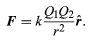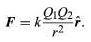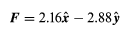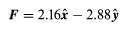Coulomb’s law

# Coulomb’s law - Notes - IIT JAM

 1 Crore+ students have signed up on EduRev. Have you?

Static electricity is a familiar electric phenomenon in which charged particles are transferred from one body to another. For example, if two objects are rubbed together, especially if the objects are insulators and the surrounding air is dry, the objects acquire equal and opposite charges and an attractive force develops between them. The object that loses electrons becomes positively charged, and the other becomes negatively charged. The force is simply the attraction between charges of opposite sign. The properties of this force were described above; they are incorporated in the mathematical relationship known as Coulomb’s law. The electric force on a charge Q1 under these conditions, due to a charge Q2 at a distance r, is given by Coulomb’s law,(1)
The bold characters in the equation indicate the vector nature of the force, and the unit vector is a vector that has a size of one and that points from charge Q2 to charge Q1. The proportionality constant k equals 10−7c2, where c is the speed of light in a vacuum; k has the numerical value of 8.99 × 109 newtons-square metre per coulomb squared (Nm2/C2). Figure 1 shows the force on Q1 due to Q2. A numerical example will help to illustrate this force. Both Q1 and Q2 are chosen arbitrarily to be positive charges, each with a magnitude of 10−6 coulomb. The charge Q1 is located at coordinates x, y, z with values of 0.03, 0, 0, respectively, while Q2 has coordinates 0, 0.04, 0. All coordinates are given in metres. Thus, the distance between Q1 and Q2 is 0.05 metre.
The magnitude of the force F on charge Q1 as calculated using equation (1) is 3.6 newtons; its direction is shown in Figure 1. The force on Q2 due to Q1 is −F, which also has a magnitude of 3.6 newtons; its direction, however, is opposite to that of F. The force F can be expressed in terms of its components along the x and y axes, since the force vector lies in the xy plane. This is done with elementary trigonometry from the geometry of Figure 1, and the results are shown in Figure 2. Thus,(1)(2)

in newtons. Coulomb’s law describes mathematically the properties of the electric force between charges at rest. If the charges have opposite signs, the force would be attractive; the attraction would be indicated in equation (1) by the negative coefficient of the unit vector r̂. Thus, the electric force on Q1 would have a direction opposite to the unit vector and would point from Q1 to Q2. In Cartesian coordinates, this would result in a change of the signs of both the x and y components of the force in equation (2).(2)

How can this electric force on Q1 be understood? Fundamentally, the force is due to the presence of an electric field at the position of Q1. The field is caused by the second charge Q2 and has a magnitude proportional to the size of Q2. In interacting with this field, the first charge some distance away is either attracted to or repelled from the second charge, depending on the sign of the first charge.

The document Coulomb’s law - Notes - IIT JAM is a part of IIT JAM category.
All you need of IIT JAM at this link: IIT JAM
 Use Code STAYHOME200 and get INR 200 additional OFF

Track your progress, build streaks, highlight & save important lessons and more!

,

,

,

,

,

,

,

,

,

,

,

,

,

,

,

,

,

,

,

,

,

;